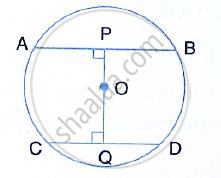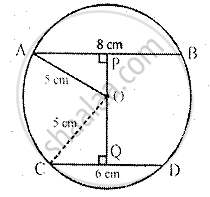Share

Books Shortlist

# In the Given Figure, O is the Centre of the Circle with Radius 5 Cm. Op and Oq Are Perpendicular to Ab and Cd Respectively. Ab = 8 Cm and Cd = 6 Cm. Determine the Length of Pq. - ICSE Class 10 - Mathematics

ConceptChord Properties - the Perpendicular to a Chord from the Center Bisects the Chord (Without Proof)

#### Question

In the given figure, O is the centre of the circle with radius 5 cm. OP and OQ are perpendicular to AB and CD respectively. AB = 8 cm and CD = 6 cm. determine the length of PQ.#### SolutionRadius of the circle whose centre is 0 = 5 cm
OP ⊥ AB and OQ ⊥ CD, AB = 8 cm and CD = 6 cm.
Join OA and OC, then OA = OC + 5 cm
Since OP ⊥ AB, P is the midpoint of AB
Similarly, Q is the midpoint of CD
In right ∆OAP,

OA^2 = OP^2 + AP^2    [Pythagoras Theorem]

⇒ (5)^2 = OP^2 + (4)^2      [ ∵ AP = PB = 1/2xx 8 = 4 cm]
⇒  25 = OP^2+16

⇒ OP^2 = 25 -16

⇒ OP^2 = 9
⇒OP = 3 cm

Similarly, in right ∆OCQ,

OC^2 = OQ^2 + CQ^2     [Pythagoras theorem]
⇒(5)^2 = OQ^2 + (3)^2

⇒25 = OQ^2 + 9

⇒ OQ^2 = 25 - 9

⇒OQ^2 = 16
⇒ OQ = 4cm

Hence, PQ = OP + OQ = 3 + 4 = 7 cm

Is there an error in this question or solution?

#### Video TutorialsVIEW ALL 

Solution In the Given Figure, O is the Centre of the Circle with Radius 5 Cm. Op and Oq Are Perpendicular to Ab and Cd Respectively. Ab = 8 Cm and Cd = 6 Cm. Determine the Length of Pq. Concept: Chord Properties - the Perpendicular to a Chord from the Center Bisects the Chord (Without Proof).
S